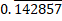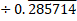# Quiz Discussion

If 2994 ÷ 14.5 = 172, then 29.94 ÷ 1.45 = ?

Course Name: Quantitative Aptitude

• 1] 0.172
• 2] 1.72
• 3] 17.2
• 4] 172
##### Solution
No Solution Present Yet

#### Top 5 Similar Quiz - Based On AI&ML

Quiz Recommendation System API Link - https://fresherbell-quiz-api.herokuapp.com/fresherbell_quiz_api

# Quiz
1
Discuss

The rational numbers lying between 1/3 and 3/4 are :

• 1]

$$\frac{{117}}{{300}},\frac{{287}}{{400}}$$

• 2]

$$\frac{{95}}{{300}},\frac{{301}}{{400}}$$

• 3]

$$\frac{{99}}{{300}},\frac{{301}}{{400}}$$

• 4]

$$\frac{{97}}{{300}},\frac{{299}}{{500}}$$

##### Solution
2
Discuss

Express 1999/2111 in decimal :

• 1] 0.893
• 2] 0.904
• 3] 0.946
• 4] 0.986
##### Solution
3
Discuss

534.596 + 61.472 - 496.708 = ? + 27.271

• 1] 62.069
• 2] 72.089
• 3] 126.631
• 4] 132.788
• 5] None of these
##### Solution
4
Discuss

617 + 6.017 + 0.617 + 6.0017 = ?

• 1] 6.2963
• 2] 62.965
• 3] 629.6357
• 4] None of these
##### Solution
5
Discuss

When 0.232323..... is converted into a fraction, then the result is:

• 1]

1/5

• 2]

2/9

• 3]

23/99

• 4]

23/100

##### Solution
6
Discuss

Which of the following are in descending order of their value ?

• 1]

$$\frac{1}{3},\frac{2}{5},\frac{3}{7},\frac{4}{5},\frac{5}{6},\frac{6}{7}$$

• 2]

$$\frac{1}{3},\frac{2}{5},\frac{3}{5},\frac{4}{7},\frac{5}{6},\frac{6}{7}$$

• 3]

$$\frac{1}{3},\frac{2}{5},\frac{3}{5},\frac{4}{6},\frac{5}{7},\frac{6}{7}$$

• 4]

$$\frac{6}{7},\frac{5}{6},\frac{4}{5},\frac{3}{7},\frac{2}{5},\frac{1}{3}$$

##### Solution
7
Discuss

32.4 × 11.5 × 8.5 = ?

• 1] 3149.5
• 2] 3129.1
• 3] 3167.1
• 4] 3162.5
• 5] None of these
##### Solution
8
Discuss

3889 + 12.952 - ? = 3854.002

• 1] 47.095
• 2] 47.752
• 3] 47.932
• 4] 47.95
##### Solution
9
Discuss

Which of the following fractions lies between 2/3 and 3/5 = ?

• 1]

2/5

• 2]

1/3

• 3]

1/15

• 4]

31/50

##### Solution
10
Discussis equal to :

• 1]

1/2

• 2]

1/3

• 3]

2

• 4]

10

# Quiz